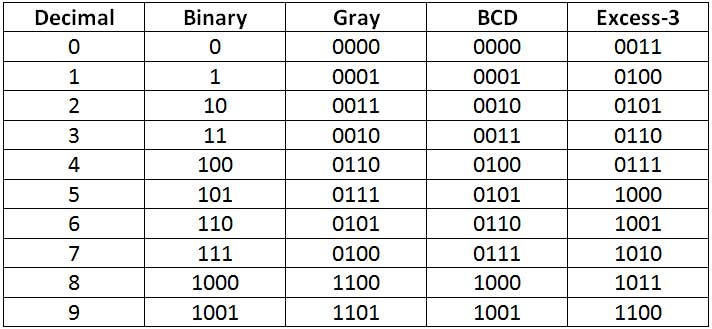# Unit 1 » Number Systems, Operations and Codes

### Types of codes

BCD (Binary-Coded Decimal) code :

• Four-bit code that represents one of the ten decimal digits from 0 to 9.
• Example – (37)10 is represented as 0011 0111 using BCD code, rather than (100101)2 in straight binary code.
• Thus BCD code requires more bits than straight binary code.
• Still it is suitable for input and output operations in digital systems.

Note: 1010, 1011, 1100, 1101, 1110, and 1111 are INVALID CODE in BCD code.

ASCII (American Standard Code Information Interchange) code :

• It is 7-bit or 8-bit alphanumeric code.
• 7-bit code is standard ASCII supports 127 characters.
• Standard ASCII series starts from 00h to 7Fh, where 00h-1Fh are used as control characters and 20h-7Fh as graphics symbols.
• 8-bit code is extended ASCII supports 256 symbols where special graphics and math’s symbols are added.
• Extended ASCII series starts from 80h to FFh.

EBCDIC (Extended Binary Coded Decimal Interchange Code) code

• 8-bit alphanumeric code developed by IBM, supports 256 symbols.
• It was mainly used in IBM mainframe computers.

Gray code

<hr/.

• Differs from leading and following number by a single bit.
• Gray code for 2 is 0011 and for 3 is 0010.
• No weights are assigned to the bit positions.
• Extensively used in shaft encoders.

Excess-3 code

• 4-bit code is obtained by adding binary 0011 to the natural BCD code of the digit.
• Example – decimal 2 is coded as 0010 + 0011 = 0101 as Excess-3 code.
• It not weighted code.
• Its self-complimenting code, means 1’s complement of the coded number yields 9’s complement of the number itself.
• Used in digital system for performing substraction operations.Table listing Binary, Gray, BCD and Express-3 Coding for Decimal DigitsConverting Binary to Gray Code –
Binary-To-Decimal Conversion
Any binary number can be converted to its decimal equivalent simply by summing together the weights of the various positions in the binary number which contain a 1.

 Binary Decimal 110112 24 + 23+01+21+20 =16+8+0+2+1 Result 2710

And

 Binary Decimal 101101012 27+06+25+24+03+22+01+20 =128+0+32+16+0+4+0+1 Result 18110

You should have noticed that the method is to find the weights (i.e., powers of 2) for each bit position that contains a 1, and then to add them up.
Decimal-To-Binary Conversion
There are 2 methods:
· Reverse of Binary-To-Decimal Method
· Repeat Division
Reverse of Binary-To-Decimal Method

 Decimal Binary 4510 =32 + 0 + 8 + 4 +0 + 1 =25+0+23+22+0+20 Result = 1011012

Repeat Division-Convert decimal to binary
This method uses repeated division by 2.
Convert 2510 to binary

 Division Remainder Binary 25/2 = 12+ remainder of 1 1 (Least Significant Bit) 12/2 = 6 + remainder of 0 0 6/2 = 3 + remainder of 0 0 3/2 = 1 + remainder of 1 1 1/2 = 0 + remainder of 1 1 (Most Significant Bit) Result 2510 = 110012

Binary-To-Octal / Octal-To-Binary Conversion

 Octal 0 1 2 3 4 5 6 7 000 001 010 011 100 101 110 111

Each Octal digit is represented by three binary digits.

Example:
100 111 0102 = (100) (111) (010)2 = 4 7 28
Repeat Division-Convert decimal to octal
This method uses repeated division by 8.
Example: Convert 17710 to octal and binary

 Division Result Binary 177/8 = 22+ remainder of 1 1 (Least Significant Bit) 22/8 = 2 + remainder of 6 6 2/8 = 0 + remainder of 2 2 (Most Significant Bit) Result 17710 = 2618 Binary = 0101100012

Example:
2AF16 = 2 x (162) + 10 x (161) + 15 x (160) = 68710
Repeat Division- Convert decimal to hexadecimal
This method uses repeated division by 16.
Example: convert 37810 to hexadecimal and binary:

 Division Result Hexadecimal 378/16 = 23+ remainder of 10 A (Least Significant Bit)23 23/16 = 1 + remainder of 7 7 1/16 = 0 + remainder of 1 1 (Most Significant Bit) Result 37810 = 17A16 Binary = 0001 0111 10102

 Hexadecimal Digit 0 1 2 3 4 5 6 7 Binary Equivalent 0000 0001 0010 0011 0100 0101 0110 0111
 Hexadecimal Digit 8 9 A B C D E F Binary Equivalent 1000 1001 1010 1011 1100 1101 1110 1111

Each Hexadecimal digit is represented by four bits of binary digit.
Example:
1011 0010 11112 = (1011) (0010) (1111)2 = B 2 F16